# Arb 2.12 released

November 29, 2017

I have finally tagged version 2.12.0 of Arb. There are many major new features: code for numerical integration, code for DFTs of complex vectors (contributed by Pascal Molin), fast evaluation of Legendre polynomials, performance optimizations for elementary functions, improvements to elliptic functions, and a few important bugfixes. A full changelog is included at the end of this post.

## Numerical integration

As discussed in depth in the most recent post, Arb now includes fast, robust and easy-to-use code for computing integrals with rigorous error bounds. A nice example is Rump's oscillatory integral $\int_0^8 \sin(x + e^x) dx$ (integrand pictured below), which Arb evaluates to 1000-digit accuracy in 1.2 seconds.## Discrete Fourier transforms

Pascal Molin contributed the acb_dft module covering computation of discrete Fourier transforms (DFTs) of complex vectors. As usual, power-of-two lengths $n = 2^k$ are the most efficient, but different FFT algorithms have been implemented to ensure that the complexity is $O(n \log n)$ even for $n$ with large prime factors. The plot shows the time to compute a length-$n$ DFT with 100-digit precision.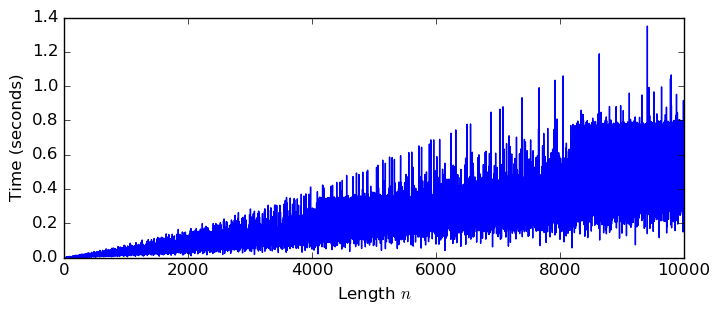With a logarithmic scale: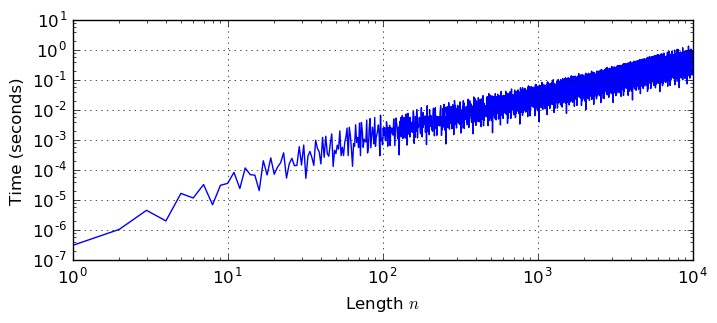There are many possible uses for DFTs, including interpolation, multipoint evaluation, and polynomial multiplication (the normal polynomial multiplication code in Arb which relies on FLINT is superior for single multiplications, but DFTs can be recycled to speed up multiplications when the same operands are used several times).

An application of DFTs in number theory is fast multi-evaluation of Dirichlet L-functions $L(s,\chi)$ for fixed $s$ over the whole group of characters $\chi$ of a given modulus $q$. The program examples/lcentral.c checks that $L(1/2,\chi)$ is nonzero for all characters $\chi$ for all $q$ in a given range. Here we run it with 128-bit precision and $q = 10^6$, which takes 9.1 seconds, where slightly more than half the time is spent computing a DFT of length $\phi(10^6) = 4 \cdot 10^5$:

build/examples/lcentral --prec 128 --check --quiet 1000000 1000000
cpu/wall(s): 9.079 9.073
virt/peak/res/peak(MB): 93.68 203.84 69.74 179.72


The current FFT code just uses straight acb_t arithmetic, so it has a lot of overhead for each arithmetic operation at low precision. With 53-bit precision, it is more than 100 times slower than an optimized double FFT library. Significant future optimization in this area is possible.

## Fast and accurate evaluation of Legendre polynomials

New code has been added for evaluating Legendre polynomials $P_n(x)$ for real $-1 \le x \le 1$ and word-size integer $n$. This code is much faster than the generic complex code used previously and gives accurate enclosures for large $n$ without requiring $O(n)$ extra precision. The improvement is significant even for moderate $n$: for example, $P_{100}([0.3 \pm 10^{-15}])$ previously took 36 microseconds and returned [0.06 +/- 4.46e-3]. It now takes 13 microseconds and returns [0.057127392203 +/- 3.44e-13]. The same $x$ with $n = 10^6$ takes 23 microseconds and returns [-0.00054506 +/- 3.16e-9] (with the old code, million-bit precision would have been needed). This is a plot of $P_{10^N+1}(x)$ for $N = 1,2,3,4,5,6$, generated at 53-bit precision: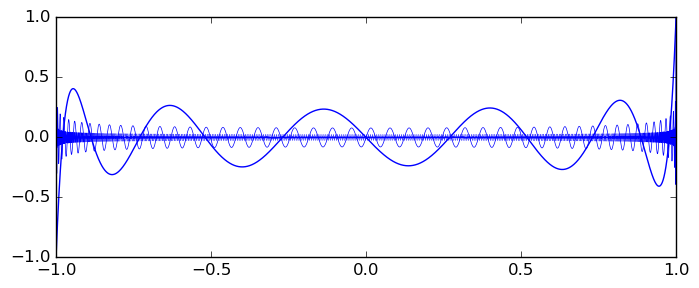Detail of $P_{10^5+1}(x)$ and $P_{10^6+1}(x)$ on $[0.9999999,1]$: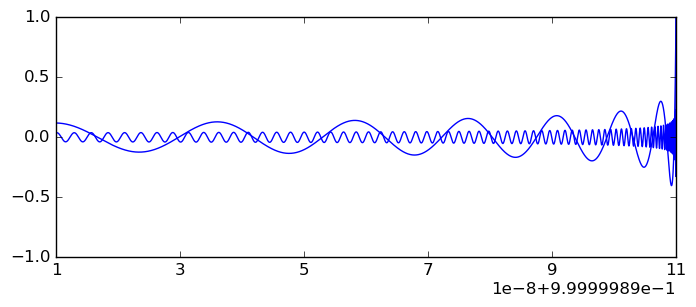There is also a new function for computing the roots of Legendre polynomials and the associated Gauss-Legendre quadrature weights (which gets called internally by the new integration code). Generating the set of roots and quadrature weights with $n = 1000$ takes 0.07 seconds at 64-bit precision and 1.3 seconds at 1000-digit precision. The degree-10000 roots and weights with 10000-digit precision take 700 seconds. At any fixed precision, the time per node is $O(1)$ when $n \to \infty$. For example, with 1000-digit precision, the whole set with $n = 10^5$ takes 221 seconds while $n = 10^6$ at the same precision takes 2016 seconds.

The new code for Legendre polynomials was developed in collaboration with Marc Mezzarobba and will be described in a forthcoming paper.

## Faster high-precision elementary functions

This was already covered in a previous post, so I just repeat the main results here. At high precision, the sine and cosine functions are now computed using custom binary splitting code instead of calling MPFR. This is up to twice as fast (near 10000 digits) and 30-40% faster asymptotically. The exponential function has also been optimized in the range from about 1400 to 6000 digits.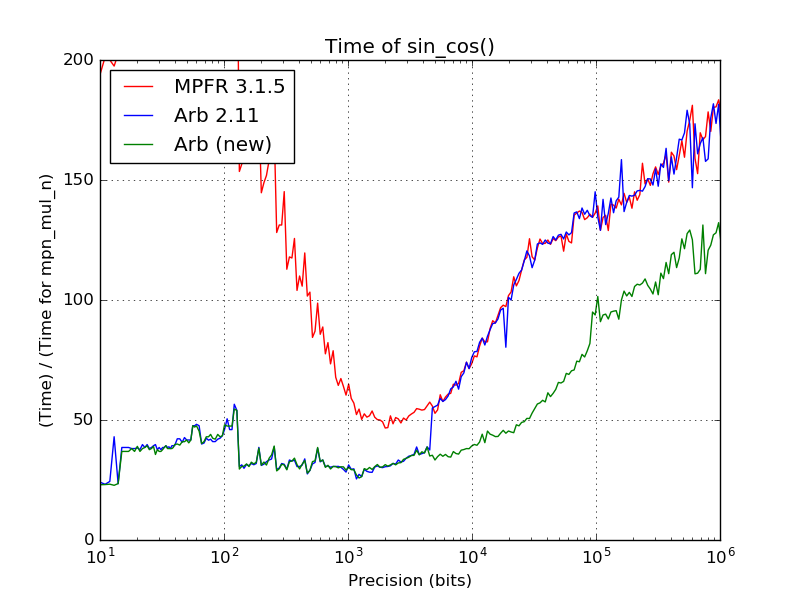## Improved elliptic functions

Several improvements have been made to elliptic and related functions:

• The algorithm for evaluating Jacobi theta functions now reduces the argument $z$ modulo the (reduced) lattice parameter $\tau$. Jacobi theta functions previously reduced the lattice parameter $\tau$ to the fundamental domain, but did not reduce the argument $z$ modulo the reduced lattice parameter. This meant that elliptic and theta functions sometimes had to be called with extremely high precision to get a useful result. For example, $\wp(100+100.25i, i)$ previously gave [+/- inf] + [+/- inf]*I at 53-bit precision and [-16.5982 +/- 6.83e-5] + [+/- 2.40e-5]*I at 2048-bit precision. It now gives [-16.598166846 +/- 6.16e-10] + [+/- 5.73e-18]*I at 53-bit precision.
• The incomplete elliptic integrals now use an algorithm with subquadratic complexity. For the incomplete elliptic integral of the first kind $F(z,m)$, the Carlson $R_F$ integral, or the inverse Weierstrass elliptic function $\wp^{-1}$, the new code is 4 times faster at 1000-digit precision and 31 times faster at 10000-digit precision. The speedup is slightly smaller for the other elliptic integrals.
• The arithmetic-geometric mean (AGM) computation now uses a longer series expansion, which results in a significant speedup (around a factor two) at low precision. In addition, the derivative of the arithmetic-geometric mean (AGM) is now computed using a central finite difference instead of a forward difference, which is 30% faster asymptotically. This in turn speeds up the complete elliptic integral of the second kind.

In related news, my slides on "Numerics of classical elliptic functions, elliptic integrals and modular forms" from a workshop at DESY in October contain some more information about the algorithms for elliptic functions.

## Full changelog

• Numerical integration
• Added a new function (acb_calc_integrate) for rigorous numerical integration using adaptive subdivision and Gauss-Legendre quadrature. This largely obsoletes the old integration code using Taylor series.
• Added new integrals.c example program (old example program moved to integrals_taylor.c).
• Discrete Fourier transforms
• Added acb_dft module with various FFT algorithm implementations, including top level O(n log n) acb_dft and acb_dft_inverse functions (contributed by Pascal Molin).
• Legendre polynomials
• Added arb_hypgeom_legendre_p_ui for fast and accurate evaluation of Legendre polynomials. This is also used automatically by the Legendre functions, where it is substantially faster and gives better error bounds than the generic algorithm.
• Added arb_hypgeom_legendre_p_ui_root for fast computation of Legendre polynomial roots and Gauss-Legendre quadrature nodes (used internally by the new integration code).
• Added arb_hypgeom_central_bin_ui for fast computation of central binomial coefficients (used internally for Legendre polynomials).
• Dirichlet L-functions and zeta functions
• Fixed a bug in the Riemann zeta function involving a too small error bound in the implementation of the Riemann-Siegel formula for inexact input. This bug could result in a too small enclosure when evaluating the Riemann zeta function at an argument of large imaginary height without also computing derivatives, if the input interval was very wide.
• Add acb_dirichlet_zeta_jet; also made computation of the first derivative of Riemann zeta function use the Riemann-Siegel formula where appropriate.
• Added acb_dirichlet_l_vec_hurwitz for fast simultaneous evaluation of Dirichlet L-functions for multiple characters using Hurwitz zeta function and FFT (contributed by Pascal Molin).
• Simplified interface for using hurwitz_precomp functions.
• Added lcentral.c example program (contributed by Pascal Molin).
• Improved error bounds when evaluating Dirichlet L-functions using Euler product.
• Elementary functions
• Faster custom implementation of sin, cos at 4600 bits and above instead of using MPFR (30-40% asymptotic improvement, up to a factor two speedup).
• Faster code for exp between 4600 and 19000 bits.
• Improved error bounds for acb_atan by using derivative.
• Improved error bounds for arb_sinh_cosh, arb_sinh and arb_cosh when the input has a small midpoint and large radius.
• Added reciprocal trigonometric and hyperbolic functions (arb_sec, arb_csc, arb_sech, arb_csch, acb_sec, acb_csc, acb_sech, acb_csch).
• Changed the interface of _acb_vec_unit_roots to take an extra length parameter (compatibility-breaking change).
• Improved arb_pow and acb_pow with an inexact base and a negative integer or negative half-integer exponent; the inverse is now computed before performing binary exponentiation in this case to avoid spurious blow-up.
• Elliptic functions
• Improved Jacobi theta functions to reduce the argument modulo the lattice parameter, greatly improving speed and numerical stability for large input.
• Optimized arb_agm by using a final series expansion and using special code for wide intervals.
• Optimized acb_agm1 by using a final series expansion and using special code for positive real input.
• Optimized derivative of AGM for high precision by using a central difference instead of a forward difference.
• Optimized acb_elliptic_rf and acb_elliptic_rj for high precision by using a variable length series expansion.
• Other
• Fixed incorrect handling of subnormals in arf_set_d.
• Added mag_bin_uiui for bounding binomial coefficients.